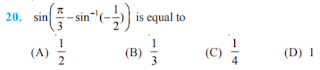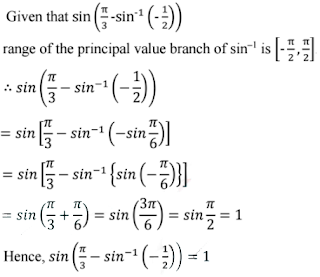#### NCERT Solutions for Class 12th: Ch 2 Inverse Trigonometric Functions Exercise 2.2 Math

Page No: 41

Exercise 2.1

Prove the following:
1. 3sin-1x = sin-1(3x – 4x3), x ∈ [-/2, 1/2]

To prove:
3sin-1x = sin-1(3x − 4x3), x ∈ [−1/2, 1/2]
Let sin-1x = θ, then x = sin θ.
We have,
RHS = sin-1(3x - 4x3)
= sin-1(3 sin θ - 4sin3θ)
= sin-1(sin 3θ) = 3θ
= 3sin-1x = LHS

2. 3cos-1x = cos-1(4x3 – 3x) x ∈ [1, 1/2]

To prove:
3cos-1x = cos-1(4x3 – 3x) x ∈ [1, 1/2].
Let cos-1x = θ, then x = cos θ.
We have,
RHS = cos-1(4x3 - 3x)
= cos-1(4cos3θ - 3cosθ)
= cos-1(cos 3θ) = 3θ
= 3cos-1x
= LHS

3. tan-12/11 + tan-17/24 = tan-11/2

To prove: tan-12/11 + tan-17/24 = tan-11/2
LHS = tan-12/11 + tan-17/24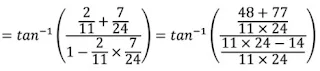= tan-1(48 + 77)/(264 − 14)
= tan-1125/250 = tan-11/2 = RHS

4. 2tan-11/2 + tan-11/7 = tan-131/17

To prove: 2tan-11/2 + tan-11/7 = tan-131/17
LHS = 2tan-11/2 + tan-11/7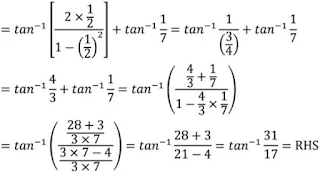Write the following functions in the simplest form:

Question: 5Question: 6Question: 7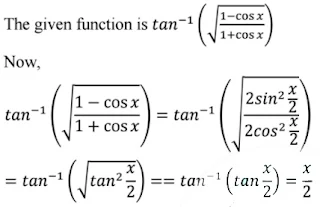Question: 8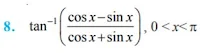Page No. 48

Question: 9Question: 10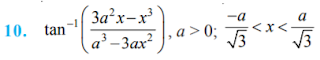Find the values of each of the following:

Question: 11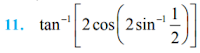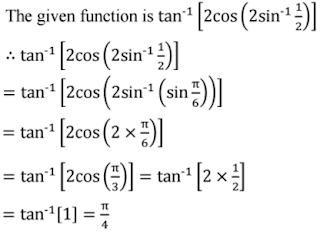Question: 12. cot (tan-1a + cot-1a)

The given function is cot(tan-1a + cot-1a).
∴ cot(tan-1a + cot-1a)
= cot (π/2) [tan-1x + cot-1x = π/2]
= 0

Question: 13Formula used:
Question: 14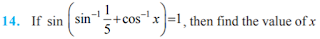Question: 15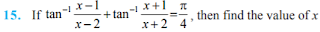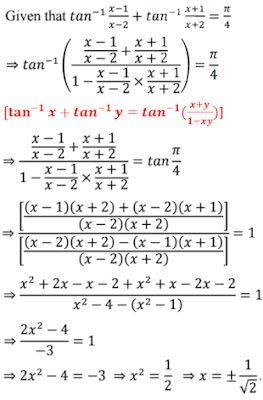Question: 16Question: 17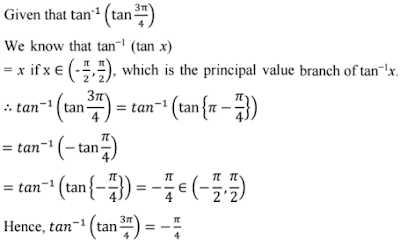Question: 18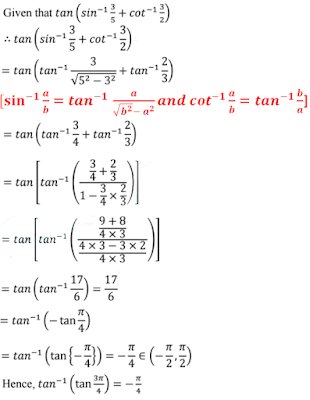Question: 19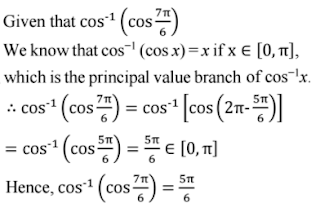The correct option is B.

Question: 20